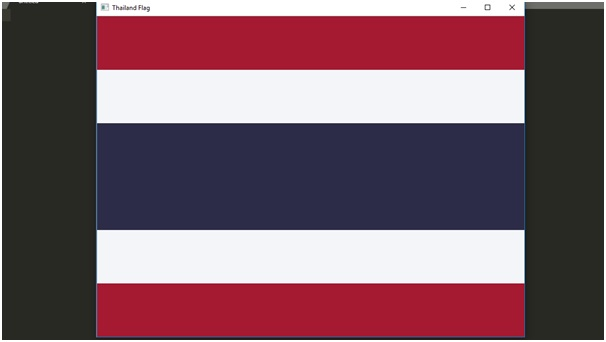# Drawing flag of Thailand | Image processing in Python

This is an example of image processing in python – here we are going to learn how to draw a flag of Thailand in python?
Submitted by Ankit Rai, on April 20, 2019

A colored image can be represented as a 3 order matrix. The first order is for the rows, the second order is for the columns and the third order is for specifying the color of the corresponding pixel. Here we use the BGR color format(because OpenCV python library works on BGR format, not on RGB), so the third order will take 3 values of Blue, Green, and Red respectively. The values of the rows and columns depending on the size of the image.

In Python programming language, we can use an OpenCV library named cv2. Python does not come with cv2, so we need to install it separately.

For Windows:

```    pip install opencv-python
```

For Linux:

```    sudo apt-get install python-opencv
```

Colour planes of BGR image:

Consider a BGR image array I then,

• I[:, :, 0] represents the Blue colour plane of the BGR image
• I[:, :, 1] represents the Green colour plane of the BGR image
• I[:, :, 2] represents the Red colour plane of the BGR image

The flag of Thailand shows five horizontal stripes in the colors red, white, blue, white and red, with the central blue stripe being twice as wide as each of the other four.

Steps:

• First, we make a matrix of dimensions 600 X 800 X 3. Where the number of pixels of rows is 600, the number of pixels of columns is 800 and 3 is the color coding in BGR format.
• Paint the first and the last strip with red. BGR is (49, 25, 165).
• Paint the middle strip with blue. BGR is (72, 44, 45).
• Paint the remaining two strips with white. BGR is (248, 245, 244).

## Python code to draw flag of Thailand

```# Python3 code to draw Thailand flag

# import numpy library as np
import numpy as np

# import open-cv library
import cv2

# here image is of class 'uint8', the range of values
# that each colour component can have is [0 - 255]

# create a zero matrix of order 600x800 of 3-dimension
image = np.zeros((600, 800, 3),np.uint8)

# Painting the Red Strip
image[:100,:,0] = 49;
image[:100,:,1] = 25;
image[:100,:,2] = 165;

# Painting the White Strip
image[100:200,:,0] = 248;
image[100:200,:,1] = 245;
image[100:200,:,2] = 244;

# Painting the Blue Strip
image[200:400,:,0] = 72;
image[200:400,:,1] = 44;
image[200:400,:,2] = 45;

# Painting the white Strip
image[400:500,:,0] = 248;
image[400:500,:,1] = 245;
image[400:500,:,2] = 244;

# Painting the Red Strip
image[500:600,:,0] = 49;
image[500:600,:,1] = 25;
image[500:600,:,2] = 165;

# Show the image formed
cv2.imshow("Thailand Flag",image);
```

OutputPreparation